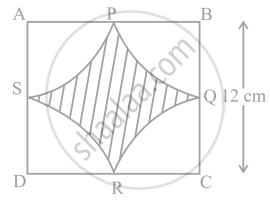# Find the area of the shaded region in figure, where arcs drawn with centres A, B, C and D intersect in pairs at mid-points P, Q, R and S of the sides AB, BC, CD and DA, respectively of a - Mathematics

Sum

Find the area of the shaded region in figure, where arcs drawn with centres A, B, C and D intersect in pairs at mid-points P, Q, R and S of the sides AB, BC, CD and DA, respectively of a square ABCD (Use π = 3.14).#### Solution

Given, side of a square BC = 12 cm

Since, Q is a mid-point of BC.

∴ Radius = BQ = 12/2 = 6 cm

Now, area of quadrant BPQ = (pir^2)/4 = (3.14 xx (6)^2)/4 = 113.04/4 cm2

Area of four quadrants = (4 xx 113.04)/4 = 1123.04 cm2

Now, area of square ABCD = (12)2 = 144 cm2

∴ Area of the shaded region = Area of square – Area of four quadrans

= 144 – 113.04

= 30.96 cm2

Concept: Areas of Combinations of Plane Figures
Is there an error in this question or solution?

#### APPEARS IN

NCERT Mathematics Exemplar Class 10
Chapter 11 Area Related To Circles
Exercise 11.3 | Q 11 | Page 127
Share